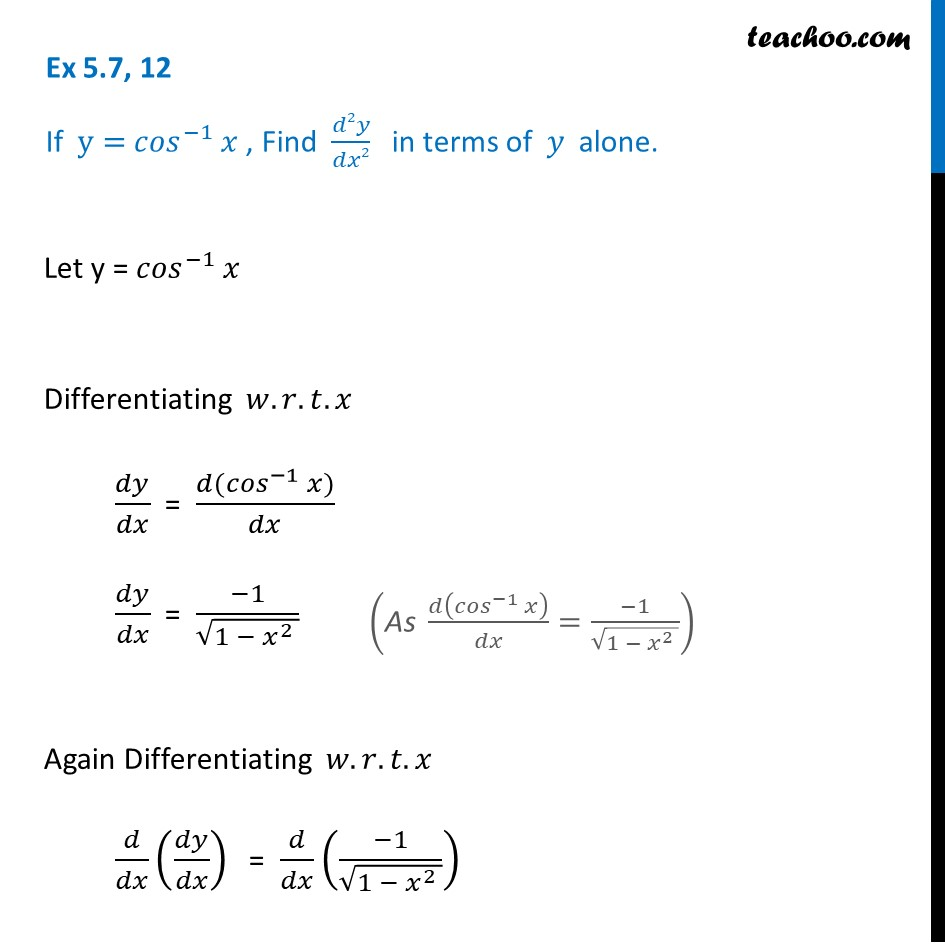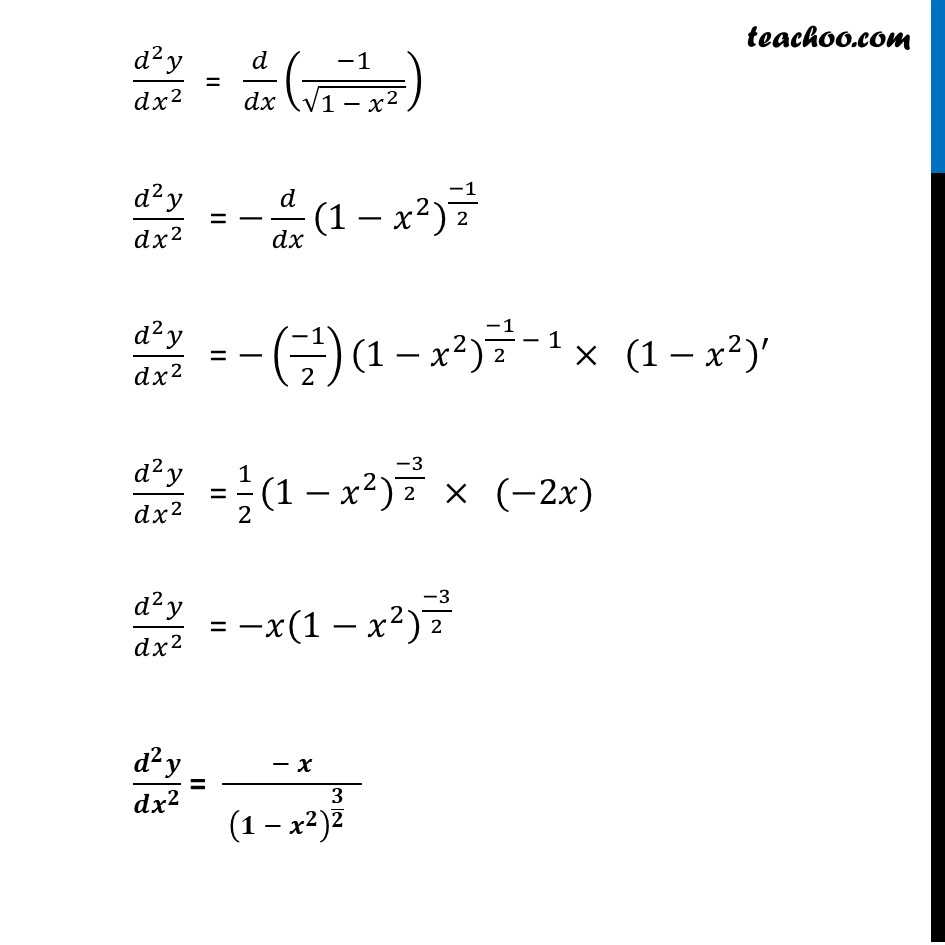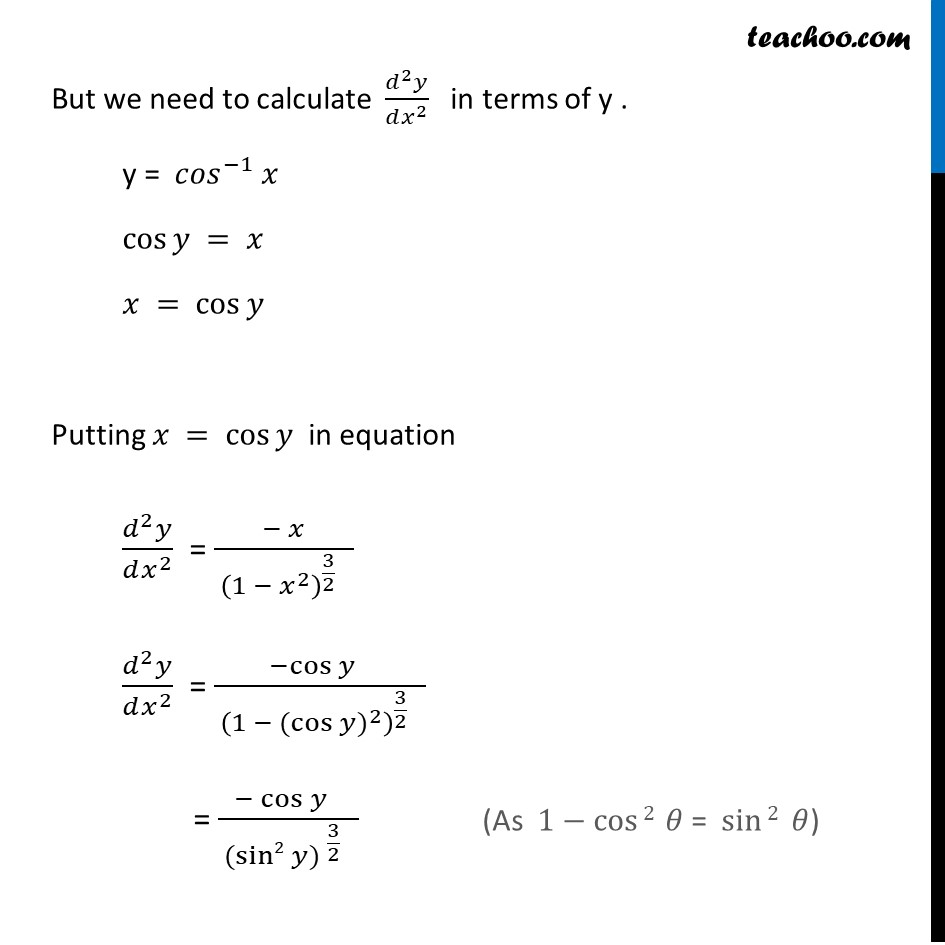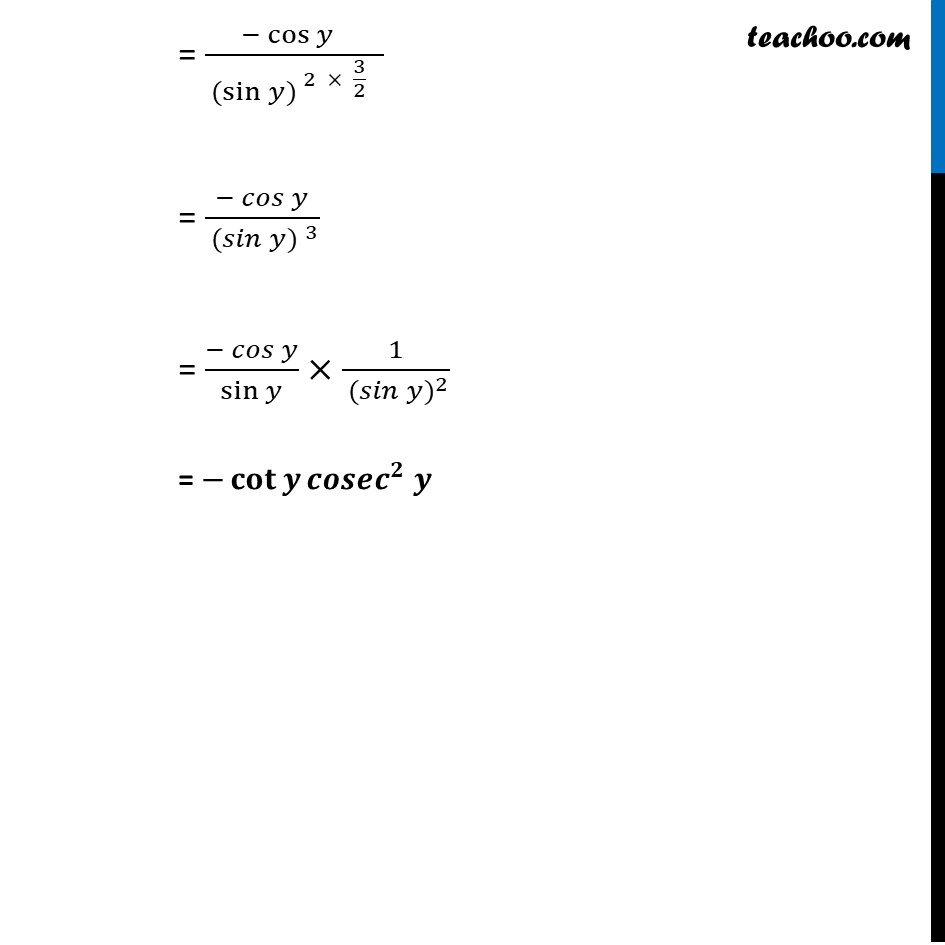Ex 5.7

Chapter 5 Class 12 Continuity and Differentiability
Serial order wiseGet live Maths 1-on-1 Classs - Class 6 to 12

### Transcript

Ex 5.7, 12 If y= 〖𝑐𝑜𝑠〗^(−1) 𝑥 , Find 𝑑2𝑦/𝑑𝑥2 in terms of 𝑦 alone.Let y = 〖𝑐𝑜𝑠〗^(−1) 𝑥 Differentiating 𝑤.𝑟.𝑡.𝑥 𝑑𝑦/𝑑𝑥 = (𝑑(〖𝑐𝑜𝑠〗^(−1) 𝑥))/𝑑𝑥 𝑑𝑦/𝑑𝑥 = (−1)/√(1 − 𝑥^2 ) Again Differentiating 𝑤.𝑟.𝑡.𝑥 𝑑/𝑑𝑥 (𝑑𝑦/𝑑𝑥) = 𝑑/𝑑𝑥 ((−1)/√(1 − 𝑥^2 )) ("As" 𝑑(〖𝑐𝑜𝑠〗^(−1) 𝑥)/𝑑𝑥=(−1)/√(1 − 𝑥^2 )) (𝑑^2 𝑦)/(𝑑𝑥^2 ) = 𝑑/𝑑𝑥 ((−1)/√(1 − 𝑥^2 )) (𝑑^2 𝑦)/(𝑑𝑥^2 ) = −𝑑/𝑑𝑥 (1−𝑥^2 )^((−1)/2) (𝑑^2 𝑦)/(𝑑𝑥^2 ) = −((−1)/2) (1−𝑥^2 )^((−1)/2 − 1)× (1−𝑥^2 )^′ (𝑑^2 𝑦)/(𝑑𝑥^2 ) = 1/2 (1−𝑥^2 )^((−3)/2) × (−2𝑥) (𝑑^2 𝑦)/(𝑑𝑥^2 ) = −𝑥(1−𝑥^2 )^((−3)/2) (𝒅^𝟐 𝒚)/(𝒅𝒙^𝟐 ) = (− 𝒙)/( (𝟏 − 𝒙^𝟐 )^(𝟑/𝟐 ) ) But we need to calculate (𝑑^2 𝑦)/(𝑑𝑥^2 ) in terms of y . y = 〖𝑐𝑜𝑠〗^(−1) 𝑥 cos⁡𝑦 = 𝑥 𝑥 = cos⁡𝑦 Putting 𝑥 = cos⁡𝑦 in equation (𝑑^2 𝑦)/(𝑑𝑥^2 ) = (− 𝑥)/( (1 − 𝑥^2 )^(3/2 ) ) (𝑑^2 𝑦)/(𝑑𝑥^2 ) = (−cos⁡𝑦 " " )/( (1 − 〖(cos⁡𝑦)〗^2 )^(3/2 ) ) = (− cos⁡𝑦 " " )/( 〖(sin2⁡〖𝑦) 〗〗^(3/2 ) ) (As 1−cos⁡〖2 〗 𝜃 = sin⁡2 𝜃) = (− cos⁡𝑦 " " )/( 〖(sin⁡〖𝑦) 〗〗^(2 × 3/2 ) ) = (− 𝑐𝑜𝑠⁡𝑦)/( 〖(𝑠𝑖𝑛⁡〖𝑦) 〗〗^3 ) = (− 𝑐𝑜𝑠⁡𝑦)/sin⁡𝑦 ×1/( 〖(𝑠𝑖𝑛⁡𝑦)〗^2 ) = −𝐜𝐨𝐭⁡𝒚 𝒄𝒐𝒔𝒆𝒄^𝟐 𝒚